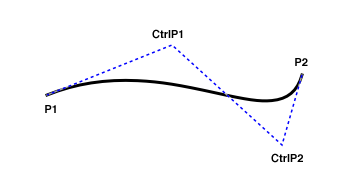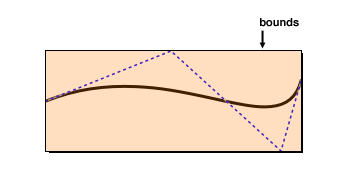# Class CubicCurve2D.Float

• `java.lang.Object`
• `java.awt.geom.CubicCurve2D`
• `java.awt.geom.CubicCurve2D.Float`
Enclosing Class:
CubicCurve2D
Implemented Interfaces:
Cloneable, Shape

`public static class CubicCurve2D.Float`
`extends CubicCurve2D`

A two-dimensional curve that is parameterized with a cubic function and stores coordinate values in single-precision floating-point format.
`CubicCurve2D.Float`

## Nested Class Summary

### Nested classes/interfaces inherited from class java.awt.geom.CubicCurve2D

`CubicCurve2D.Double`, `CubicCurve2D.Float`

## Field Summary

` float`
`ctrlx1`
The x coordinate of the curve’s first control point.
` float`
`ctrlx2`
The x coordinate of the curve’s second control point.
` float`
`ctrly1`
The y coordinate of the curve’s first control point.
` float`
`ctrly2`
The y coordinate of the curve’s second control point.
` float`
`x1`
The x coordinate of the curve’s start point.
` float`
`x2`
The x coordinate of the curve’s end point.
` float`
`y1`
The y coordinate of the curve’s start point.
` float`
`y2`
The y coordinate of the curve’s end point.

## Constructor Summary

`Float()`
Constructs a new CubicCurve2D that stores its coordinate values in single-precision floating-point format.
`Float(float x1, float y1, float cx1, float cy1, float cx2, float cy2, float x2, float y2)`
Constructs a new CubicCurve2D that stores its coordinate values in single-precision floating-point format, specifying the initial position of each point.

## Method Summary

` Rectangle2D`
`getBounds2D()`
Determines the smallest rectangle that encloses the curve’s start, end and control points.
` Point2D`
`getCtrlP1()`
Returns the curve’s first control point.
` Point2D`
`getCtrlP2()`
Returns the curve’s second control point.
` double`
`getCtrlX1()`
Returns the x coordinate of the curve’s first control point.
` double`
`getCtrlX2()`
Returns the s coordinate of the curve’s second control point.
` double`
`getCtrlY1()`
Returns the y coordinate of the curve’s first control point.
` double`
`getCtrlY2()`
Returns the y coordinate of the curve’s second control point.
` Point2D`
`getP1()`
Returns the curve’s start point.
` Point2D`
`getP2()`
Returns the curve’s end point.
` double`
`getX1()`
Returns the x coordinate of the curve’s start point.
` double`
`getX2()`
Returns the x coordinate of the curve’s end point.
` double`
`getY1()`
Returns the y coordinate of the curve’s start point.
` double`
`getY2()`
Returns the y coordinate of the curve’s end point.
` void`
`setCurve(double x1, double y1, double cx1, double cy1, double cx2, double cy2, double x2, double y2)`
Changes the curve geometry, separately specifying each coordinate value as a double-precision floating-point number.
` void`
`setCurve(float x1, float y1, float cx1, float cy1, float cx2, float cy2, float x2, float y2)`
Changes the curve geometry, separately specifying each coordinate value as a single-precision floating-point number.

### Methods inherited from class java.awt.geom.CubicCurve2D

`clone`, `contains`, `contains`, `contains`, `contains`, `getBounds`, `getCtrlP1`, `getCtrlP2`, `getCtrlX1`, `getCtrlX2`, `getCtrlY1`, `getCtrlY2`, `getFlatness`, `getFlatness`, `getFlatness`, `getFlatnessSq`, `getFlatnessSq`, `getFlatnessSq`, `getP1`, `getP2`, `getPathIterator`, `getPathIterator`, `getX1`, `getX2`, `getY1`, `getY2`, `intersects`, `intersects`, `setCurve`, `setCurve`, `setCurve`, `setCurve`, `setCurve`, `solveCubic`, `solveCubic`, `subdivide`, `subdivide`, `subdivide`

### Methods inherited from class java.lang.Object

`clone`, `equals`, `extends Object> getClass`, `finalize`, `hashCode`, `notify`, `notifyAll`, `toString`, `wait`, `wait`, `wait`

## Field Details

### ctrlx1

`public float ctrlx1`
The x coordinate of the curve’s first control point.

### ctrlx2

`public float ctrlx2`
The x coordinate of the curve’s second control point.

### ctrly1

`public float ctrly1`
The y coordinate of the curve’s first control point.

### ctrly2

`public float ctrly2`
The y coordinate of the curve’s second control point.

### x1

`public float x1`
The x coordinate of the curve’s start point.

### x2

`public float x2`
The x coordinate of the curve’s end point.

### y1

`public float y1`
The y coordinate of the curve’s start point.

### y2

`public float y2`
The y coordinate of the curve’s end point.

## Constructor Details

### Float

`public Float()`
Constructs a new CubicCurve2D that stores its coordinate values in single-precision floating-point format. All points are initially at position (0, 0).

### Float

```public Float(float x1,
float y1,
float cx1,
float cy1,
float cx2,
float cy2,
float x2,
float y2)```
Constructs a new CubicCurve2D that stores its coordinate values in single-precision floating-point format, specifying the initial position of each point.Parameters:
`x1` - the x coordinate of the curve’s start point.
`y1` - the y coordinate of the curve’s start point.
`cx1` - the x coordinate of the curve’s first control point.
`cy1` - the y coordinate of the curve’s first control point.
`cx2` - the x coordinate of the curve’s second control point.
`cy2` - the y coordinate of the curve’s second control point.
`x2` - the x coordinate of the curve’s end point.
`y2` - the y coordinate of the curve’s end point.

## Method Details

### getBounds2D

`public Rectangle2D getBounds2D()`
Determines the smallest rectangle that encloses the curve’s start, end and control points. As the illustration below shows, the invisible control points may cause the bounds to be much larger than the area that is actually covered by the curve.Specified by:
getBounds2D in interface Shape

### getCtrlP1

`public Point2D getCtrlP1()`
Returns the curve’s first control point.
Overrides:
getCtrlP1 in interface CubicCurve2D

### getCtrlP2

`public Point2D getCtrlP2()`
Returns the curve’s second control point.
Overrides:
getCtrlP2 in interface CubicCurve2D

### getCtrlX1

`public double getCtrlX1()`
Returns the x coordinate of the curve’s first control point.
Overrides:
getCtrlX1 in interface CubicCurve2D

### getCtrlX2

`public double getCtrlX2()`
Returns the s coordinate of the curve’s second control point.
Overrides:
getCtrlX2 in interface CubicCurve2D

### getCtrlY1

`public double getCtrlY1()`
Returns the y coordinate of the curve’s first control point.
Overrides:
getCtrlY1 in interface CubicCurve2D

### getCtrlY2

`public double getCtrlY2()`
Returns the y coordinate of the curve’s second control point.
Overrides:
getCtrlY2 in interface CubicCurve2D

### getP1

`public Point2D getP1()`
Returns the curve’s start point.
Overrides:
getP1 in interface CubicCurve2D

### getP2

`public Point2D getP2()`
Returns the curve’s end point.
Overrides:
getP2 in interface CubicCurve2D

### getX1

`public double getX1()`
Returns the x coordinate of the curve’s start point.
Overrides:
getX1 in interface CubicCurve2D

### getX2

`public double getX2()`
Returns the x coordinate of the curve’s end point.
Overrides:
getX2 in interface CubicCurve2D

### getY1

`public double getY1()`
Returns the y coordinate of the curve’s start point.
Overrides:
getY1 in interface CubicCurve2D

### getY2

`public double getY2()`
Returns the y coordinate of the curve’s end point.
Overrides:
getY2 in interface CubicCurve2D

### setCurve

```public void setCurve(double x1,
double y1,
double cx1,
double cy1,
double cx2,
double cy2,
double x2,
double y2)```
Changes the curve geometry, separately specifying each coordinate value as a double-precision floating-point number.Overrides:
setCurve in interface CubicCurve2D
Parameters:
`x1` - the x coordinate of the curve’s new start point.
`y1` - the y coordinate of the curve’s new start point.
`cx1` - the x coordinate of the curve’s new first control point.
`cy1` - the y coordinate of the curve’s new first control point.
`cx2` - the x coordinate of the curve’s new second control point.
`cy2` - the y coordinate of the curve’s new second control point.
`x2` - the x coordinate of the curve’s new end point.
`y2` - the y coordinate of the curve’s new end point.

### setCurve

```public void setCurve(float x1,
float y1,
float cx1,
float cy1,
float cx2,
float cy2,
float x2,
float y2)```
Changes the curve geometry, separately specifying each coordinate value as a single-precision floating-point number.Parameters:
`x1` - the x coordinate of the curve’s new start point.
`y1` - the y coordinate of the curve’s new start point.
`cx1` - the x coordinate of the curve’s new first control point.
`cy1` - the y coordinate of the curve’s new first control point.
`cx2` - the x coordinate of the curve’s new second control point.
`cy2` - the y coordinate of the curve’s new second control point.
`x2` - the x coordinate of the curve’s new end point.
`y2` - the y coordinate of the curve’s new end point.